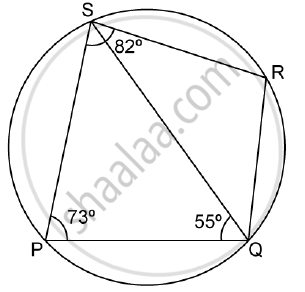Share

# Pqrs is a Cyclic Quadrilateral. Given ∠Qps = 73°, ∠Pqs = 55° and ∠Psr = 82°, Calculate: - Mathematics

Course

#### Question

PQRS is a cyclic quadrilateral. Given ∠QPS = 73°, ∠PQS = 55° and ∠PSR = 82°, calculate:

1) ∠QRS

2) ∠RQS

3) ∠PRQ#### Solution

Given: PQRS is a cyclic quadrilateral.

∠QPS = 73°, ∠PQS = 55° and ∠PSR = 82°

1) Opposite angle of a cyclic quadrilateral is supplementary.

⇒ ∠QPS + ∠QRS = 180°

⇒ 73° +  ∠QRS = 180°

⇒ ∠QRS = 180° - 73= 107°

2) Opposite angle of a cyclic quadrilateral are supplementary.

⇒ ∠PSR + ∠PQR = 180°

⇒ ∠PSR + (⇒ ∠PQS + ⇒ ∠RQS) = 180°

⇒ 82° + 55° + ∠RQS = 180°

⇒ ∠RQS = 180° - 137° = 43°

3) In ΔPQS, by angle sum property, we have

⇒ ∠PSQ + ∠PQS + ∠QPS = 180°

⇒ ∠PSQ + 55° + 73° = 180°

⇒ ∠PSQ = 180° - 128° = 52°

Now ⇒ ∠PRQ = ∠PSQ  (angles in the same segment of a circle)

⇒ ∠PRQ = 52°

Is there an error in this question or solution?